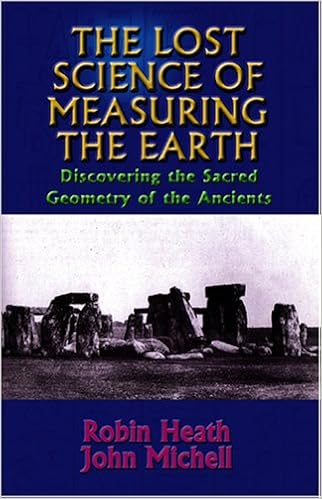# Ten Fingers set the Limits for Counting for the HonestTen Fingers set the Limits for Counting for the Honest. I reference Henry David Thoreau.  Our life is frittered away by detail. An honest man has hardly need to count more than his ten fingers. On extreme cases he may add his ten toes, and lump the rest. Simplicity, simplicity, simplicity! Henry David ThoreauWALDEN: Or, Life in the Woods.

The numbers 1 – 5 were basic in ancient measure. The ancients were even more simplistic than stated in the quote by  Thoreau. You could count the fingers on one hand to understand ancient  sacred philosophy. Here are but of examples of ancient math using the 1st five numbers.

• Our first example uses the same total as numbers one through five squared:  Please follow the process: Total triple straight combinations on the 3 x 3 number square that total 15. This is done by three boxes in straight lines: First here is the product of the 1st five numbers:  1 x 2 x 3 x 4 x 5  = 120. On the square find 8 separate combinations of numbers totaling 15: Three are horizontal. Three are vertical. Two are diagonal:  Horizontal first: 4 + 9 + 2 = 15. Next, 3 + 5 + 7  = 15. Then, 8 + 1 + 6 = 15. Next we tally vertical combinations: 4 + 3 + 8 = 15. Next, 9 + 5 + 1 = 15. Then, 2 + 7 + 6 = 15. Diagonal combinations are:  4 + 5 + 6 = 15. Then 2+ 5 + 8 =15.  Thus, 45 + 45 + 30 = 120. Again, this is the same total as product of the numbers 1 – 5.
•The primary unit of distance measure around the world was the megalithic mile. It was 14,400 feet. John Michell and Robin Heath amply cover this unit in their writings,Square numbers 1 – 5 and multiply them.  The product equals a basic measure of antiquity by number. The megalithic mile of  14,400 feet.

Again, look at the 1st five numbers.  1²  x 2² x 3² x 4² x 5² = 14,400. Of course, the basic numbers of the Pythagorean right triangle are the 3, 4, 5. . It states tthe square of the hypotenuse (the side opposite the right angle) is equal to the sum of the squares of the other two sides. Thus, 3 ² + 4² = 5².Pythagorean theorem
The sum of the areas of the two squares on the legs (a and b) equals the area of the hypotenuse.

### We Hardly Need Even Ten Fingers for Counting

Let get even more basic: 1 ² + 2² = 5. That defines the center of the 3 x 3 number square. There are  five regular polyhedrons called the Platonic Solids. If you say so what? The pattern continues:  Take numbers 2 and 3:  We see that in likewise fashion 2² + 3² = 13. On the 5 x 5 number square, 13 is the central number. Thirteen also defines the number of semi-regular solids. See the internal link below for pictures of the 7 basic number squares of antiquity.
To end this blog, I reiterate the three words by Thoreau:  Simplicity, simplicity, simplicity! Even ten fingers are too many.  Why simplicity? It can help us regain a lost Golden Age of peace and plenty.